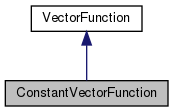Chombo + EB + MF  3.2
ConstantVectorFunction Class Reference

`#include <ConstantVectorFunction.H>`

Inheritance diagram for ConstantVectorFunction:[legend]

## Public Member Functions

ConstantVectorFunction (const RealVect &a_value)

~ConstantVectorFunction ()
Destructor. More...

RealVect operator() (const RealVect &a_x, Real a_t) const

RealVect derivative (const IntVect &a_order, const RealVect &a_x, Real a_t) constPublic Member Functions inherited from VectorFunction
VectorFunction (bool a_homogeneous, bool a_constant)

virtual ~VectorFunction ()
Destructor. More...

virtual bool hasDerivative (const IntVect &a_order) const

RealVect operator() (const RealVect &a_x) const

RealVect derivative (const IntVect &a_order, const RealVect &a_x) const

bool isHomogeneous () const
Returns true if this function is homogeneous, false otherwise. More...

bool isConstant () const
Returns true if this function is constant, false otherwise. More...

## Private Attributes

RealVect m_valueProtected Attributes inherited from VectorFunction
bool m_isHomogeneous

bool m_isConstant

## Detailed Description

This VectorFunction subclass represents a function that returns a constant value everywhere in space and time.

## ◆ ConstantVectorFunction()

 ConstantVectorFunction::ConstantVectorFunction ( const RealVect & a_value )
explicit

Creates a constant scalar function returning the given value.

Parameters
 a_value The value returned by the function.

## ◆ ~ConstantVectorFunction()

 ConstantVectorFunction::~ConstantVectorFunction ( )

Destructor.

## ◆ operator()()

 RealVect ConstantVectorFunction::operator() ( const RealVect & a_x, Real a_t ) const
virtual

Override this method to evaluate this function at the given point in space and time.

Parameters
 a_x A point in-dimensional space. a_t The time at which the function is evaluated.

Implements VectorFunction.

## ◆ derivative()

 RealVect ConstantVectorFunction::derivative ( const IntVect & a_order, const RealVect & a_x, Real a_t ) const
virtual

Override this method to evaluate the given partial derivative of the function at the given point in space and time.

Parameters
 a_order A multi-index identifying the order(s) of the partial derivative of the function to be evaluated. a_x A point in-dimensional space. a_t The time at which the derivative is to be evaluated.

Reimplemented from VectorFunction.

## ◆ m_value

 RealVect ConstantVectorFunction::m_value
private

The documentation for this class was generated from the following file: